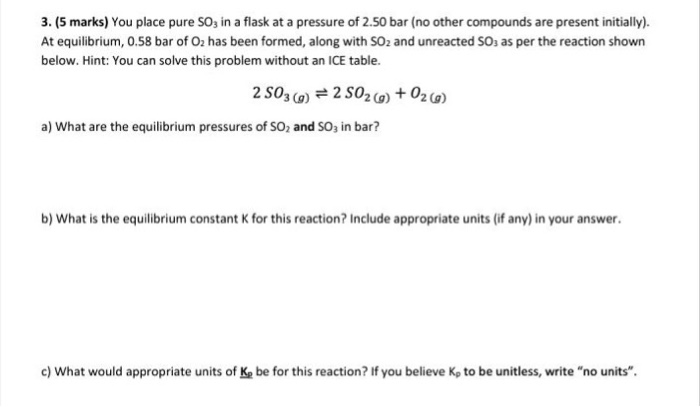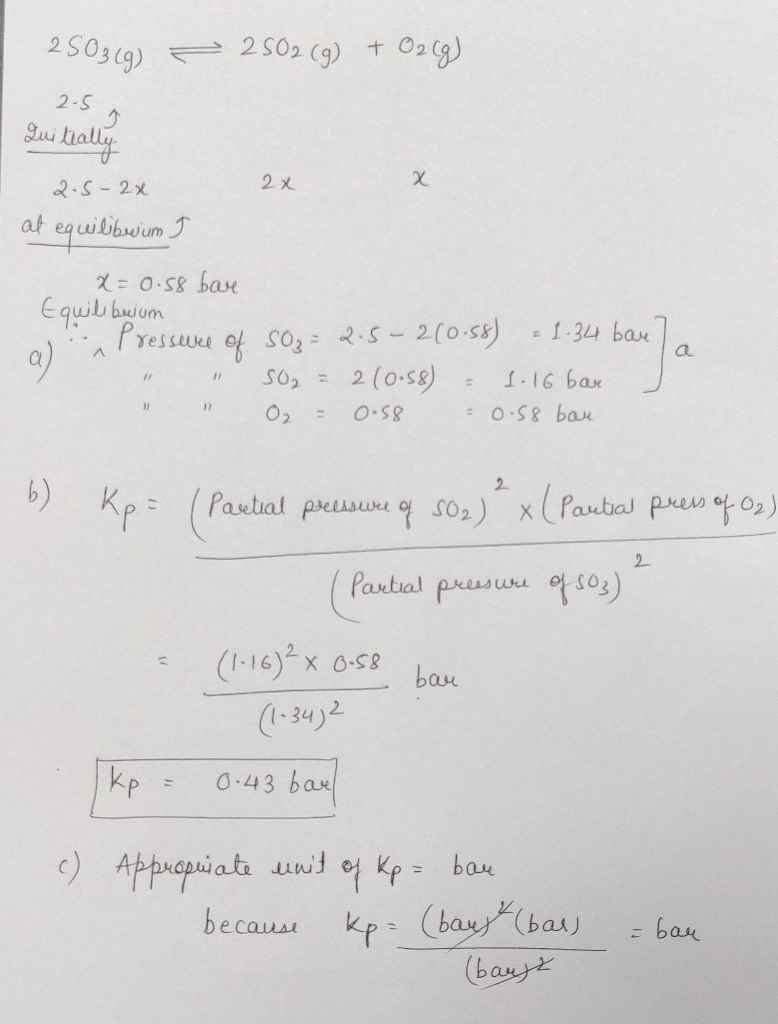Homework Help Question & Answers

# 3. (5 marks) You place pure SO3 in a flask at a pressure of 2.50 bar (no other compounds are pres...3. (5 marks) You place pure SO3 in a flask at a pressure of 2.50 bar (no other compounds are present initially). At equilibrium, 0.58 bar of O has been formed, along with SO2 and unreacted SOs as per the reaction shown below. Hint: You can solve this problem without an ICE table. 3(g) 2 SO2(g a) What are the equilibrium pressures of SO; and SO, in bar? b) What is the equilibrium constant K for this reaction? Include appropriate units (if any) in your answer. c) What would appropriate units of Ke be for this reaction? If you believe Kp to be unitless, write "no units"##### Add Answer of: 3. (5 marks) You place pure SO3 in a flask at a pressure of 2.50 bar (no other compounds are pres...
More Homework Help Questions Additional questions in this topic.

• #### At a certain temperature, 0.840 mol of SO3 is placed in a 1.50-L container. At eqibrium, 0.140 mol of O2 is present. Calculate Kc Number K= At a certain temperature, 0.840 mol of SO3 is placed i...

Need Online Homework Help?

Get FREE EXPERT Answers
WITHIN MINUTES
Related Questions